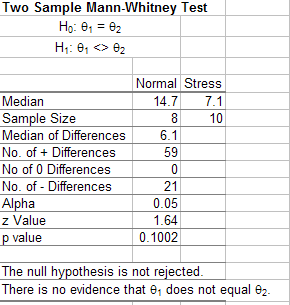Need to find something out about samples when you have a very small sample size or the data are not normally distributed?  The answer is to use nonparametric testing.

• Does the median equal a certain value?
• Are the medians of my control group and test group different?
• Which of these four teaching methods is best?

## Nonparametric Methods and SPC for Excel

SPC for Excel contains four nonparametric methods. Many statistical methods are based on the assumption of normally distributed data. This assumption is often not valid or the sample size is too small to determine if the data are normally distributed. Parametric tests do not require the data to be normally distributed. These tests are sometimes called distribution-free methods. The output for one of the methods (Mann-Whitney) is shown above.

• One Sample Sign Test
• One Sample Wilcoxon Sign Test
• Mann-Whitney Test for Two Samples
• Kruskal-Wallis Test for Multiple Samples